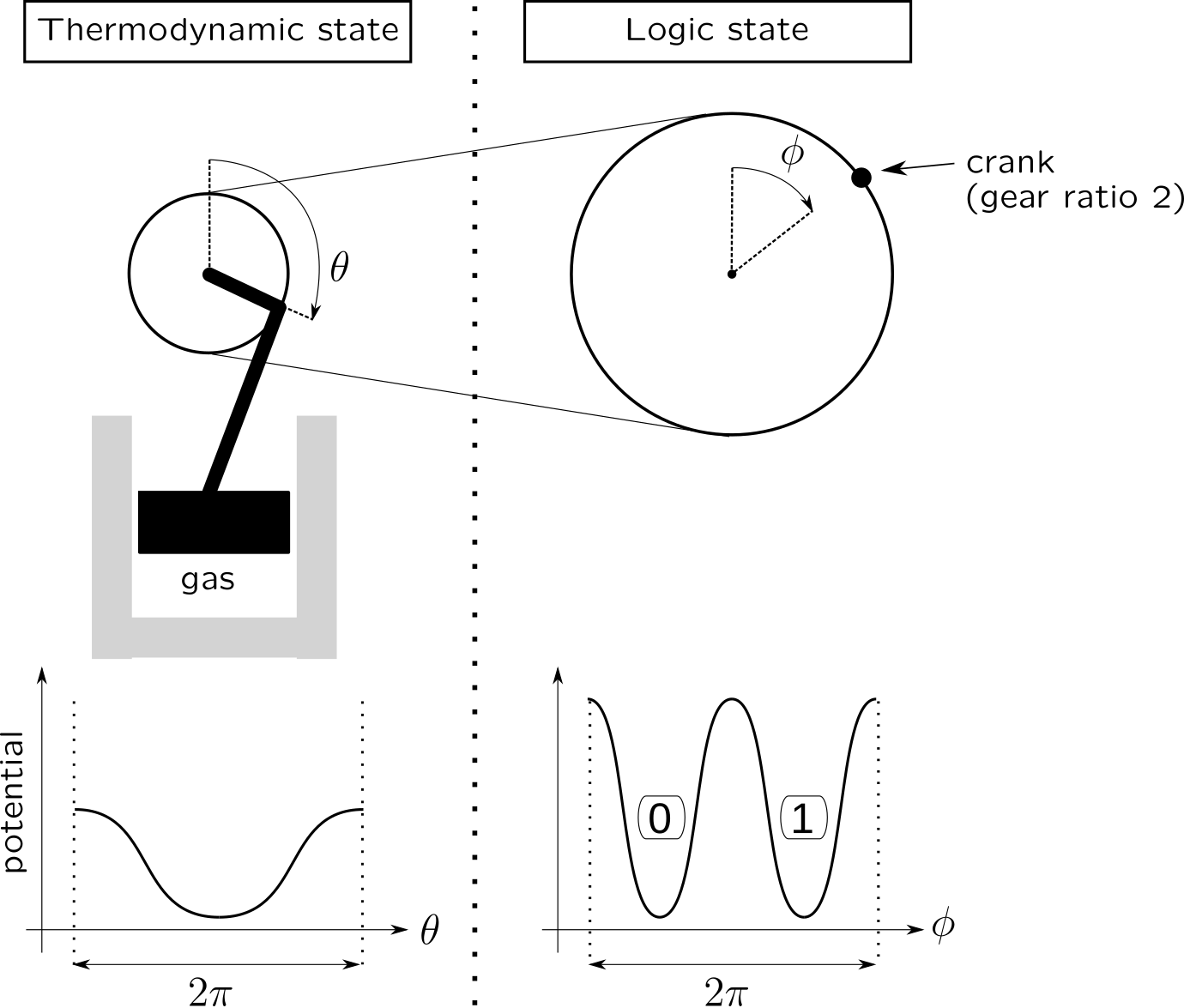# Thermodynamical versus logical irreversibility : a concrete objection to Landauer's principle

Landauer’s principle states that the logical irreversibility of an operation, such as erasing one bit, whatever its physical implementation, necessarily implies its thermodynamical irreversibility.

In this paper, a very simple counterexample of physical implementation (that uses a two-to-one relation between logic and thermodynamic states) is given that allows to erase one bit in a thermodynamical quasistatic manner (i.e. that may tend to be reversible if slowed down enough).Two-to-one implementation of a bit inspired by my practice of bicycles: the two bit-values correspond to one single thermodynamic state. Erasing the bit (setting it to 0) is necessarily thermodynamically reversible.